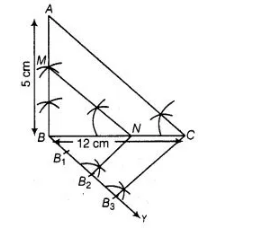# Draw a right ΔABC in which BC = 12 cm,

Question:

Draw a right ΔABC in which BC = 12 cm, AB = 5 cm and ∠B = 90°.Construct a triangle similar to it and of scale factor Is the new triangle also a right

triangle?

Solution:

Steps of construction

1. Draw a line segment BC = 12 cm,
2. From 6 draw a line AB = 5 cm which makes right angle at B.3. Join $A C, \triangle A B C$ is the given right triangle.

4. From $B$ draw an acute $\angle C B Y$ downwards.

5. On ray BY, mark three points $B_{1}, B_{2}$ and $B_{3}$, such that $B B_{1}=B_{1} B_{2}=B_{2} B_{3}$.

6. Join $B_{3} C$.

7. From point $B_{2}$ draw $B_{2} N \| B_{3} C$ intersect $B C$ at $N$.

8. From point $N$ draw $N M \| C A$ intersect $B A$ at $M . \triangle M B N$ is the required triangle. $\triangle M B N$ is also a right angled triangle at $B$.